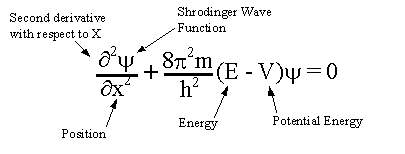# Brief Intro to Partial Differential EquationsMathematical modeling is an important tool used by engineers and physicists to understand and predict the behavior of complex systems. It allows us to take real-world problems and translate them into mathematical equations that can be solved and analyzed. One powerful tool used in mathematical modeling is the partial differential equation (PDE).
Before diving into PDEs, it is helpful to have a basic understanding of calculus and ordinary differential equations (ODEs). Calculus is a branch of mathematics that deals with the study of rates of change and accumulation. It is the foundation of mathematical modeling and is used to represent real-world problems in mathematical terms. ODEs are a type of calculus equation that describes the rate of change of a quantity over time. They are used to model many physical phenomena, such as the motion of a ball thrown into the air or the flow of water in a river.
PDEs, on the other hand, are used to model systems that involve multiple variables and multiple dimensions. They are used to describe the behavior of systems that change over both space and time. PDEs are used to model a wide range of physical phenomena, including heat transfer, fluid flow, and electromagnetic fields.
There are many types of PDEs, but some of the most commonly used are:
-The Laplace equation, which is used to describe steady-state heat transfer, electrostatics, and fluid flow.
-The Poisson equation, which is used to describe the distribution of charge in a system.
-The Navier-Stokes equations, which are used to describe the motion of fluids.
-The Heat equation, which describes the diffusion of heat in a system.
-The Schrödinger equation, which is used in quantum mechanics to describe the behavior of particles.
Solving PDEs involves finding a mathematical function that satisfies the equation and the boundary conditions of the problem. The solution to a PDE is a function that represents the behavior of the system being modeled. There are many methods for solving PDEs, but some of the most commonly used include separation of variables, the method of characteristics, and numerical methods such as finite difference and finite element methods.
In reality, PDEs have a wide range of applications. In the field of engineering, PDEs are used to design and analyze heat exchangers, aircraft wings, and other structures. In the field of physics, PDEs are used to study the behavior of fluids, such as the flow of water in a river or the motion of air over an aircraft wing. In the field of finance, PDEs are used to model the behavior of stock prices and option prices. In the field of biology, PDEs are used to model the spread of diseases and the movement of species.
In conclusion, PDEs are an important tool used by engineers and physicists to model complex systems that change over both space and time. They are used to describe a wide range of physical phenomena and are used in many fields such as engineering, physics, finance, and biology. Understanding the basics of calculus and ODEs and familiarizing with some common types of PDEs, their solutions and applications can give a general audience valuable insight into the power and importance of mathematical modeling in understanding the world around us.